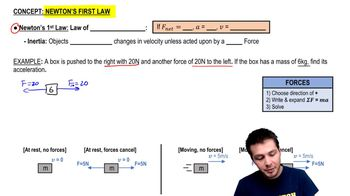Start typing, then use the up and down arrows to select an option from the list.
3:30 minutes
Problem 4c
Textbook Question

# A 4.50-kg experimental cart undergoes an acceleration in a straight line (the x-axis). The graph in Fig. E4.13 shows this acceleration as a function of time. (a) Find the maximum net force on this cart. When does this maximum force occur? (image)Verified Solution
This video solution was recommended by our tutors as helpful for the problem above.
123views